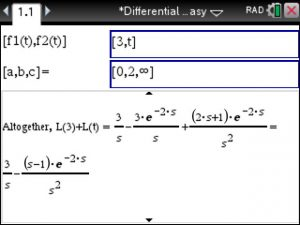## TiNSpire CX: Solve System of Differential Equations using LaPlace Transform – Step by Step

Say you have to solve the system of Differential Equations shown in below’s image. Launch the Differential Equations Made Easy app (download at www.TiNspireApps.com) , go to Laplace Transforms in the menu and just type in as shown below:

Scrolling down to view all steps finally shows the correct final answer:

Posted on Categories differential equation, laplace transform

## TiNspire CX CAS : Solve Differential Equations – Step by Step – using LaPlace Transforms

Using the Differential Equations Made Easy APP at https://tinspireapps.com/?a=deqme you can solve Differential Equations using LaPlace Transforms as shown below:

Posted on Categories differential equation, laplace transform

## TiNspire: LaPlace Transforms of a Piecewise-Defined Function

Laplace transform over Piecewise def. Function

Example:

f(1) = 3 defined over 0<= t <2

f(2) = t  defined over t >= 2

To find the LaPlace Transform use Differential Equations Made Easy at

https://www.tinspireapps.com/?a=DEQME  and select LAPLACE TRANSFORM OF PIECEWISE DEFINED FUNCTION and enter as follows:We use infinity since the function f2 is not bounded. If it was bounded by for example 10 then we would have entered as [0,2,10]

Posted on Categories differential equation, laplace transform